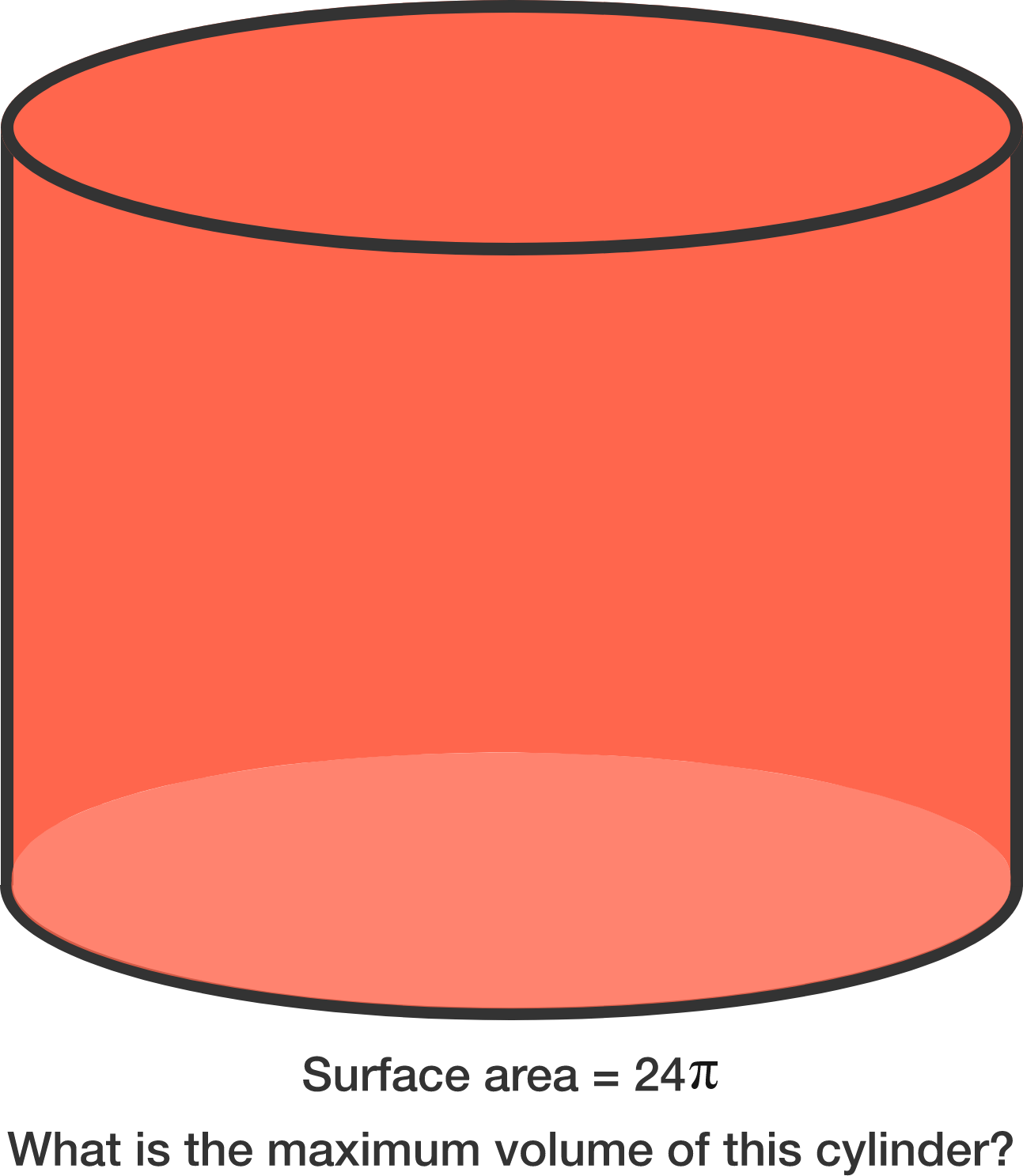# Maximizing Volume Given Closed Area

Calculus Level 3If $V\pi$ is the maximum possible volume of a closed cylinder such that its surface area is $24\pi$, what is the value of $V$?

×